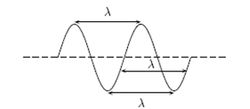# WavelengthFigure 1 Wavelength is measured from anywhere on the wave until the shape repeats

The wavelength of a specific wave is the distance over which a wave repeats, as shown in Figure 1. These repeating patterns known as wavelengths are represented by the letter lambda (λ). Waves carry energy through a medium (like the atmosphere, the vacuum, or even the ocean). For more on the physics of waves please see hyperphysics.

Light is categorized according to its wavelength or frequency. The frequency f, wavelength λ, and speed v of the wave are connected by the equation v = λf. For light, c is the speed of light, and the equation becomes c = λf. The longer the wavelength, the lower the energy for the photon (the packet of light); due to the equation for energy contained in a photon:

or 

Where:

As shown in Figure 2, the wavelength of light goes from smaller than an atom to the size of cities!

When light comes in from the sun and strikes the surface of the Earth, the light bounces back and the wavelength gets longer. This longer wavelength light (now in the infrared) interacts with the greenhouse gases in the atmosphere and bounces around, warming the planet. This difference in wavelength is important because the shorter wavelength photons coming in pass right through the greenhouse gases (like carbon dioxide, methane, or water vapour), but the longer wavelength (lower energy) photons get absorbed. This phenomenon, known as the greenhouse effect, leads to global warming, a form of climate change.

## Phet Simulation

The University of Colorado has graciously allowed us to use the following Phet simulation. The simulation below allows you to see the interaction among speed, wavelength, and frequency. Wiggle the wrench up and down to create waves of different wavelengths. In order to see this best, click "no end," "oscillate," and turn the damping down to zero.